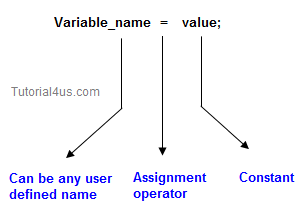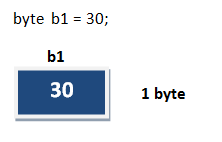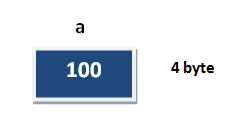# Variable in Java

## Variable Declaration Rules in Java

Variable is an identifier which holds data or another one variable is an identifier whose value can be changed at the execution time of program. Variable is an identifier which can be used to identify input data in a program.## Syntax

```Variable_name = value;
```

## Rules to declare a Variable

• Every variable name should start with either alphabets or underscore ( _ ) or dollar ( \$ ) symbol.
• No space are allowed in the variable declarations.
• Except underscore ( _ ) no special symbol are allowed in the middle of variable declaration
• Variable name always should exist in the left hand side of assignment operators.
• Maximum length of variable is 64 characters.
• No keywords should access variable name.

Note: Actually a variable also can start with ¥,¢, or any other currency sign.

## Example of Variable Declaration

```class Sum
{
public static void main(String[] args)
{
int _a, ¢b, ¥c, \$d, result;
_a=10;
¢b=20;
¥c=30;
\$d=40;
result=_a+¢b+¥c+\$d;
System.out.println("Sum is :" +result);
}
}
```

## Output

```Sum is : 100
```

## Variable initialization

It is the process of storing user defined values at the time of allocation of memory space.## Variable assignment

Value is assigned to a variable if that is already declared or initialized.

## Syntax

```	Variable_Name = value
int a = 100;
```## Syntax

```int  a= 100;
int b;
b = 25;  //  ------>  direct assigned variable
b = a;    //  ------>  assigned value in term of variable
b = a+15;  //  ------>  assigned value as term of expression
```